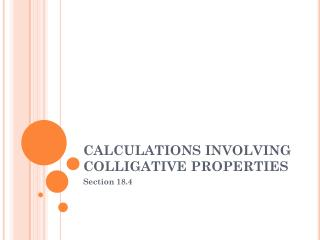DownloadDownload PresentationCALCULATIONS INVOLVING COLLIGATIVE PROPERTIES

# CALCULATIONS INVOLVING COLLIGATIVE PROPERTIES

Télécharger la présentation## CALCULATIONS INVOLVING COLLIGATIVE PROPERTIES

- - - - - - - - - - - - - - - - - - - - - - - - - - - E N D - - - - - - - - - - - - - - - - - - - - - - - - - - -
##### Presentation Transcript

1. CALCULATIONS INVOLVING COLLIGATIVE PROPERTIES Section 18.4

2. AFTER READING SECTION 18.4, YOU SHOULD KNOW: • How to calculate molality (m) • How to calculate the molar mass of a molecular compound from the freezing point depression or boiling point elevation

3. MOLALITY • Molality (m) – the number of moles of solvent dissolved per kilogram of solvent • m = mol solute / kg solvent • Mole fraction nA = moles of solute A nB moles of solvent B • XA = nA XB = nB nA + nB nA + nB

4. BOILING POINT ELEVATION • Change in boiling point temperature • ΔTb = Kb * m • ΔTb = change in boiling pt temp • Kb = molal boiling-point elevation constant • Depends on the solvent • Units (oC / m) • m = molality (mole / kg)

5. FREEZING POINT DEPRESSION • Change in freezing point temperature • ΔTf = Kf * m • ΔTf = change in freezing pt temp • Kf = molal freezing-point depression constant • Depends on the solvent • Units (oC / m) • m = molality (mole / kg)

6. AFTER READING SECTION 18.4, YOU SHOULD KNOW: • How to calculate molality (m) • How to calculate the molar mass of a molecular compound from the freezing point depression or boiling point elevation